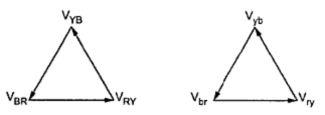### Delta-Delta Connection of 3-Phase Transformer

In this type of connection, both the three phase primary and secondary windings are connected in delta as shown in the Fig. 1.Fig.  1

The voltages on primary and secondary sides can be shown on the phasor diagram as shown in the Fig. 1(a).Fig. 1(a

The another way of representing this type of connection is shown in the Fig. 2.Fig.  2

This connection proves to be economical for large low voltage transformers as it increases number of turns per phase.
Key point : It can be seen that there is no phase shift between primary and secondary voltages.
VL1 = Line voltage on primary side.
VL2 = Line voltage on secondary side.
Vph1 = phase voltage on primary side.
Vph2 = Phase voltage on secondary side.
K = Transformer ratio.
For delta connection,    VL1 = Vph1
Now since                    Vph2 /Vph1 = K
...                                   Vph2 = K Vph1
But again since secondary is connected in delta
VL2 = Vph2 = K VL1
The advantages and disadvantages of this type of connection can be summerized as follows.
Advantages
1. in order to get secondary voltage as sinusoidal, the magnetizing current of transformer must contain a third harmonic component. The delta connection provides a closed path for circulation of third harmonic component of current. The flux remains sinusoidal which results in sinusoidal voltages.
2. Even if the load is unbalanced the three phase voltages remains constant. Thus it allows unbalanced loading also.
3. The important advantage with this type of connection is that if there is bank of single phase transformers connected in delta-delta fashion and if one of the transformers is disabled then the supply can be continued with remaining tow transformers of course with reduced efficiency.
4. There is no distortion in the secondary voltages.
5. Due to delta connection, phase voltage is same as line voltage hence winding have more number of turns. But phase current is (1/√3) times the line current. Hence the cross-section of the windings is very less. This makes the connection economical for low voltages transformers.
Disadvantages
1. Due to the absence of neutral point it is not suitable for three phase four wire system.
Key point : The connection is commonly used for large low voltage transformers.